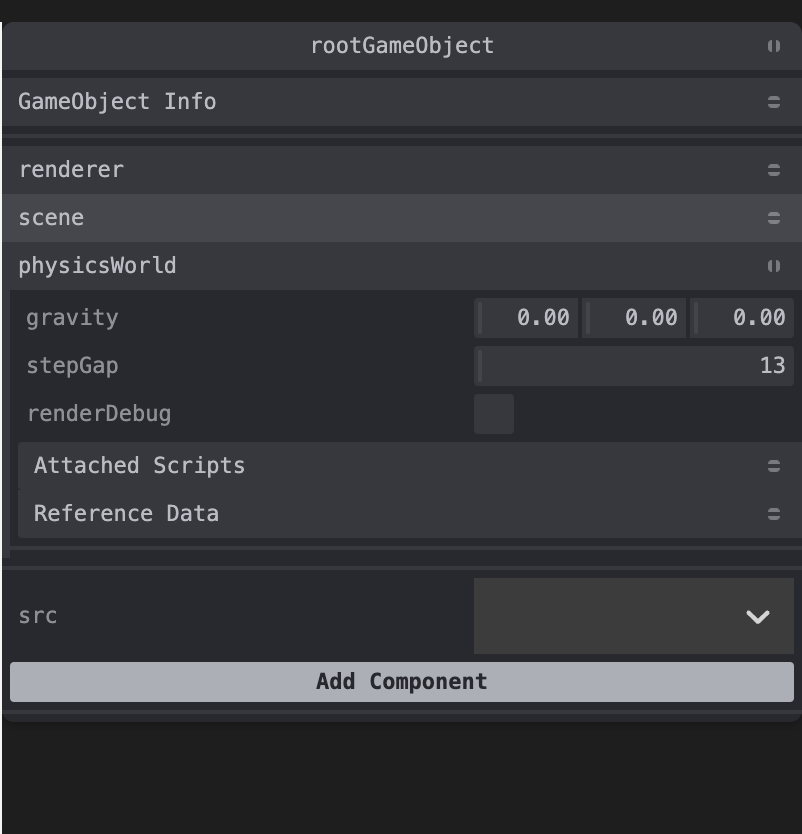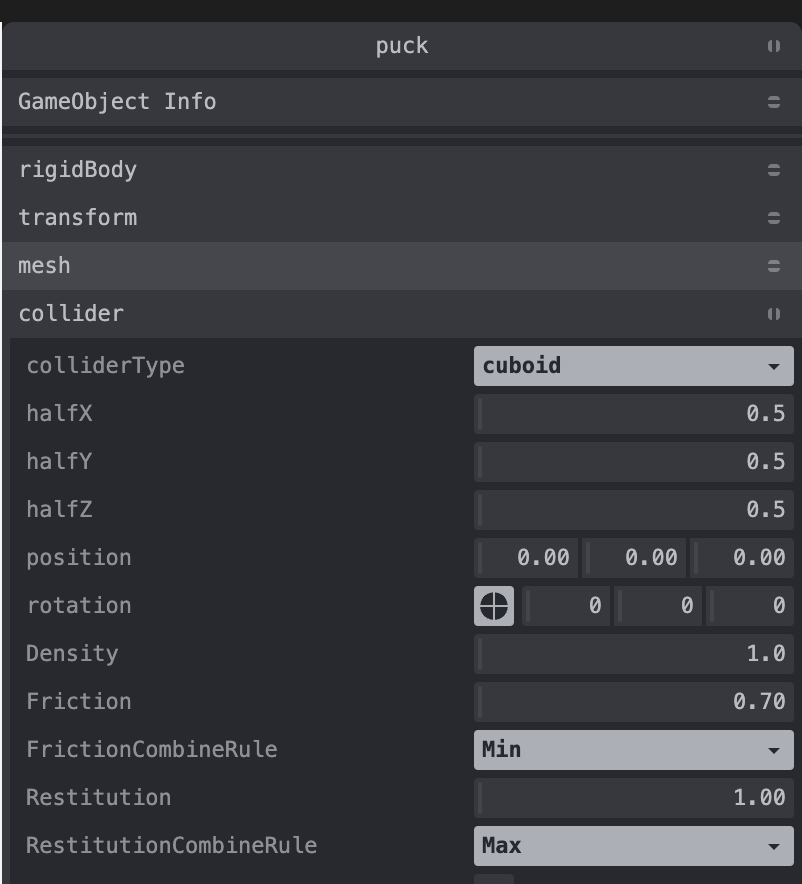# Puck ​

In this part of the tutorial we will make the puck move.

## Setup ​

1. Remove gravity from the physicsWorld component in the rootGameObject.2. Move the puck to [0,8,0]

3. Make the `Restitution`: `1` and `RestitutionCombineRule`: `Max` on the collider for the puck.## Logic ​

1. Create the file `<projectDir>/src/scripts/puck.ts` with the following code.
TypeScript
``````export const props = {
speed: 10,
}

// this event is triggered because of the rigidBody component
export const rigidBodyInitialized = (game, gameObject, {props}, rigidBody) => {
// select some random linear velocity
let linVel = {x: Math.random(), y: Math.random(), z: Math.random()*-5} as any;

const updateSpeed = () => { // ensure there is no slow down
if(!linVel)linVel = rigidBody.linvel();

const factor = (props.speed || 10)/Math.sqrt(linVel.x*linVel.x + linVel.y*linVel.y + linVel.z*linVel.z);
linVel.x *= factor;
linVel.y *= factor;
linVel.z *= factor;

if(factor === 0){linVel = {x: 0, y: 0, z: props.speed}}

rigidBody.setLinvel(linVel);
linVel = undefined;
}

Although we can set friction to 0 and expect no loss of energy as the puck bounces, linear velocity is still not conserved as the energy from velocity converts to rotational energy on bounces. Even this can be prevented by locking the puck's rotation, but letting the puck rotate lead to more random collisions, which makes the game more fun. So to compensate we ensure in side the `updateSpeed` function that speed remains unchanged.